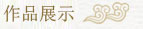»­ہبت×ز³،،،،»­ہب¼ٍ½é،،،،أû¼ز¹ْ»­،،،،أû¼زتé·¨،،،،خؤ·؟سأئ·،،،،أû¼زءذ±ي،،،،حئ¼ِأû¼ز،،،،»­ہبس°¼¯،،،،سراéء´½س،،،،ءھدµ·½ت½

 ،،ؤْµ±ا°µؤخ»ضأ£؛حّص¾ت×ز³ > تé·¨×÷ئ· ،،،،،،،،،،،،،،،،،،،،،،،،،،،،،،،،،،،،،،،،،،،،،،،،،،،،،،،،،،،،،،،،،،،،،،،،،،،،،،،،،،،،،،،،،،،،،،،،،،،،،،،،
 ،،،،،،±à؛إ£؛100405 ،،،،،،×÷صك£؛½ًَأسê ،،،،،،ئ·أû£؛تé·¨تض¾ي ،،،،،،³ك´ç£؛105x20 ،،،،،،×´ج¬£؛خ´تغ
 ،،،،،،±à؛إ£؛100404 ،،،،،،×÷صك£؛½ًَأسê ،،،،،،ئ·أû£؛تé·¨ ،،،،،،³ك´ç£؛34x34 ،،،،،،×´ج¬£؛خ´تغ
 ،،،،،،±à؛إ£؛100403 ،،،،،،×÷صك£؛½ًَأسê ،،،،،،ئ·أû£؛تé·¨ ،،،،،،³ك´ç£؛34x34 ،،،،،،×´ج¬£؛خ´تغ
 ،،،،،،±à؛إ£؛100402 ،،،،،،×÷صك£؛½ًَأسê ،،،،،،ئ·أû£؛تé·¨ ،،،،،،³ك´ç£؛33x33 ،،،،،،×´ج¬£؛خ´تغ
 ،،،،،،±à؛إ£؛100401 ،،،،،،×÷صك£؛½ًَأسê ،،،،،،ئ·أû£؛تé·¨ ،،،،،،³ك´ç£؛33x33 ،،،،،،×´ج¬£؛خ´تغ
 ،،،،،،±à؛إ£؛100400 ،،،،،،×÷صك£؛½ًَأسê ،،،،،،ئ·أû£؛تé·¨ ،،،،،،³ك´ç£؛43x32 ،،،،،،×´ج¬£؛خ´تغ
 ،،،،،،±à؛إ£؛100399 ،،،،،،×÷صك£؛½ًَأسê ،،،،،،ئ·أû£؛تé·¨ ،،،،،،³ك´ç£؛33x33 ،،،،،،×´ج¬£؛خ´تغ
 ،،،،،،±à؛إ£؛100398 ،،،،،،×÷صك£؛½ًَأسê ،،،،،،ئ·أû£؛تé·¨ذؤ¾­ ،،،،،،³ك´ç£؛110x20 ،،،،،،×´ج¬£؛خ´تغ
 ،،،،،،±à؛إ£؛100397 ،،،،،،×÷صك£؛½ًَأسê ،،،،،،ئ·أû£؛تé·¨ ،،،،،،³ك´ç£؛66x33 ،،،،،،×´ج¬£؛خ´تغ
 ،،،،،،±à؛إ£؛100396 ،،،،،،×÷صك£؛½ًَأسê ،،،،،،ئ·أû£؛تé·¨ ،،،،،،³ك´ç£؛138x69 ،،،،،،×´ج¬£؛خ´تغ
 ،،،،،،±à؛إ£؛100395 ،،،،،،×÷صك£؛½ًَأسê ،،،،،،ئ·أû£؛تé·¨ ،،،،،،³ك´ç£؛33x33 ،،،،،،×´ج¬£؛خ´تغ
 ،،،،،،±à؛إ£؛100394 ،،،،،،×÷صك£؛½ًَأسê ،،،،،،ئ·أû£؛تé·¨ ،،،،،،³ك´ç£؛33x33 ،،،،،،×´ج¬£؛خ´تغ